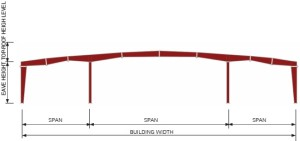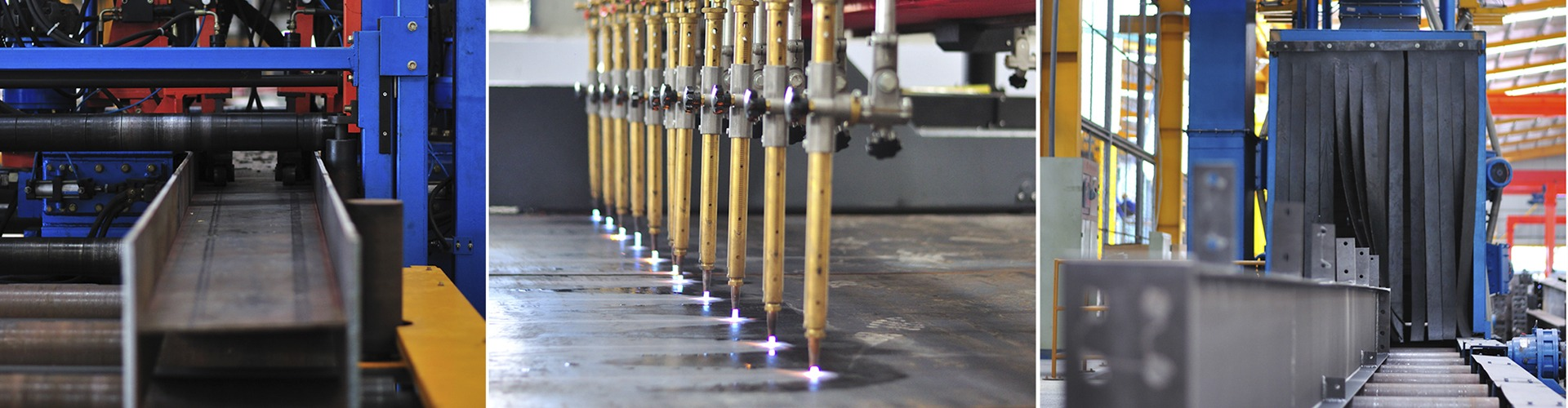# Standard Framing System

ATAD Steel Structure Corporation provides 10 usual kinds of standard framing system. Each one has its own advantages and suited for different applications.

These are standard kinds of buildings, all specific requirements are carefully considered by our technicians to best satisfy the usage needs and investment efficiency of customers.

 MAX PRACTICAL WIDTH W = 100m CS FRAME SAMPLE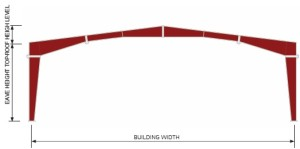MAX PRACTICAL WIDTH W = 150m MS1 FRAME SAMPLE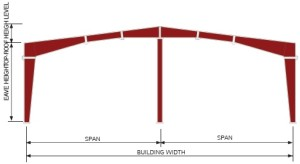MAX PRACTICAL WIDTH W = 60m SS FRAME SAMPLE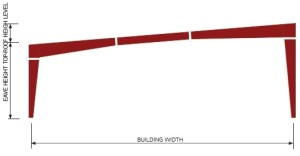MAX PRACTICAL WIDTH W = 200m MS2 FRAME SAMPLE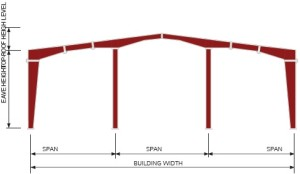MAX PRACTICAL WIDTH W = 50m RS1 FRAME SAMPLE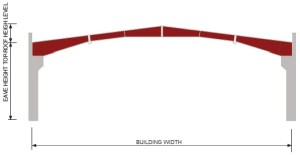MAX PRACTICAL WIDTH W = 400m MS3 FRAME SAMPLE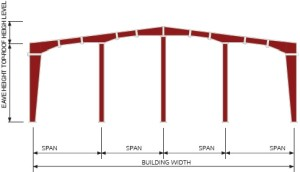MAX PRACTICAL WIDTH W = 60m RS2 FRAME SAMPLE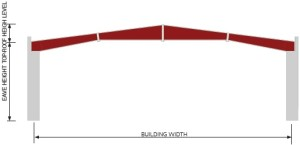MAX PRACTICAL WIDTH W = 150m MG1 FRAME SAMPLE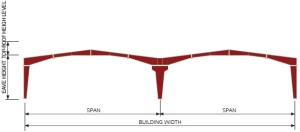MAX PRACTICAL WIDTH W = 100m CS2 FRAME SAMPLE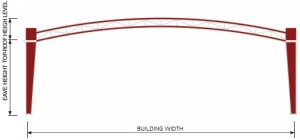MAX PRACTICAL WIDTH W = 200m CS1 FRAME SAMPLE